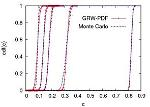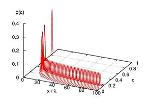Stochastic modeling of transport processes in porous mediaOrganisationseinheit:
 → Lehrstuhl für Angewandte Mathematik

FAU Kontaktperson:
 → Prechtel, Alexander Dr. → Suciu, Nicolae Dr.

Beschreibung:

The hydraulic conductivity in highly heterogeneous porous such as
natural groundwater systems can be efficiently parameterized, mainly in
large scale problems, merely as a space random function. Consequently,
the modelled concentration transported in groundwater is random and
stochastic predictive tools are required. The aim of our group is to
develop robust numerical algorithms to solve evolution equations for the
probability density function (PDF) of the random concentration field.
This involves the formulation of the PDF equation for specific transport
problems, solving large ensembles of transport problems which will be
used for parameter calibrations and as reference stochastic solutions,
and, finally, solving the PDF equation. The latter uses consistency
constraints which render the PDF equation equivalent to a Fokker-Planck
equation and to its Itô process representation. The systems of the
resulting Itô equations is solved by tracking computational particles in
physical and concentration spaces with a global random walk algorithm
(GRW), stable, free of numerical diffusion, and insensitive to the
increase of the number of particles.

Zuletzt aktualisiert 2019-22-01 um 18:03